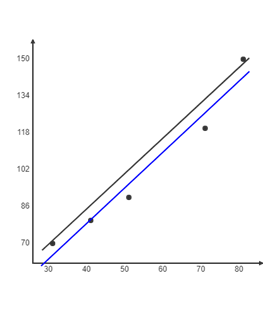top of pageSearch

# Simple and Multiple Linear RegressionLinear regression is one of the well known and well understood algorithms in statistics and machine learning. It is one of the machine learning algorithms based on supervised learning. Linear regression is a statistical model that shows the relationship between two variables with the linear equation.

## When Do You Need Regression?

You will need regression to answer whether and how some factors influence the other or how the variables are related. For example, you can use it to determine to what extent the experience or gender impact salaries.

Regression is also useful when you want to forecast the feature. For example, you could try to predict electricity consumption of a household for the next hour given the outdoor temperature, time of day, and number of residents in that household.

Regression is used in many different fields: economy, computer science, social sciences, and so on.

## Regression Analysis

Regression analysis is a form predictive modelling technique which builds the relationship between two or independent variable and dependent variables.

For example, Let’s say you want to predict the house price and that will depend on various factors like plot size, number of bedrooms, year it is build and so on...

The dependent features are called dependent variables or outputs.

The independent features are called independent variables or inputs.## What is Linear regression

Linear regression in simple term is answering a question on “How can I use X to predict Y?” where X is some information that you have, and Y is some information that you want.

Let’s say you wanted a sell a house and you wanted to know how much you can sell it for. You have information about the house that is your X and the selling price that you wanted to know will be your Y.

Linear regression creates an equation in which you input your given numbers (X) and it outputs the target variable that you want to find out (Y).

## Linear Regression model representation

Linear regression is such a useful and established algorithm, that it is both a statistical model and a machine learning model. Linear regression tries a draw a best fit line that is close to the data by finding the slope and intercept.

Linear regression equation is,

Y=a+bx

In this equation:

• y is the output variable. It is also called the target variable in machine learning or the dependent variable.

• x is the input variable. It is also referred to as the feature in machine learning or it is called the independent variable.

• a is the constant

• b is the coefficient of independent variable

Let’s take an example and see how simple linear regression works

Let’s say we need to predict our electricity bill. The electricity bill will totally depend upon the weather.Here the weather is given in Fahrenheit and Electricity bill is in dollars...

Lets plot in graph and find the best fit lineIntercept:

18.488372

Slope:

1.546512

Line of Best Fit:

y = 1.546512x + 18.488372.

## Multiple linear regression

Multiple Linear Regression assumes there is a linear relationship between two or more independent variables and one dependent variable.

The Formula for multiple linear regression:

Y=B0+B0X1+B2X2+……+BnXn+e

• Y = the predicted value of the dependent variable

• B0 = the y-intercept (value of y when all other parameters are set to 0)

• B1X1= the regression coefficient (B1) of the first independent variable (X1)

• BnXn = the regression coefficient of the last independent variable

• e = model error

The multiple linear regression model can be represented as a plane (in 2-dimensions) or a hyperplane (in higher dimensions).